Journal of Vibroengineering

Published: 20 January 2023

# Analysis of longitudinal and torsional vibration of mining scraper conveyor under the over bending section working condition

Chunxue Xie1
Zhixiang Liu2
Miao Xie3
1School of Mechanics and Engineering, Liaoning Technical University, Fuxin, China
2, 3Research Institute of Mineral Resources Development and Utilization Technology and Equipment, Liaoning Technical University, Fuxin, China
Corresponding Author:
Chunxue Xie
Views 48

#### Abstract

Considering that the scraper will produce longitudinal and torsional pendulum vibration when passing through the bending section, and have a great influence on the motion characteristics of the scraper conveyor, this paper studies the longitudinal and torsional pendulum coupling vibration characteristics of the scraper conveyor under the working condition of over-bending section for the first time. Firstly, the simplified model of torsional pendulum vibration of scraper chain system is established based on Voigt model and point-by-point tension method. Then, the change law of scraper group vibration and chain tension under the condition of scraper bending is studied. Thirdly, through the influence area and attenuation characteristics of torsional pendulum vibration, the thumbnail model of torsional pendulum vibration is constructed. Finally, the longitudinal and torsional pendulum coupling vibration dynamic model of the scraper chain system is constructed based on the torsional pendulum vibration abbreviation model, and the overall longitudinal and torsional pendulum vibration characteristics of the scraper conveyor are studied when the scraper is over the bending section. The conclusion of this paper provides a theoretical basis for better understanding the operation characteristics of scraper conveyor in coal mine.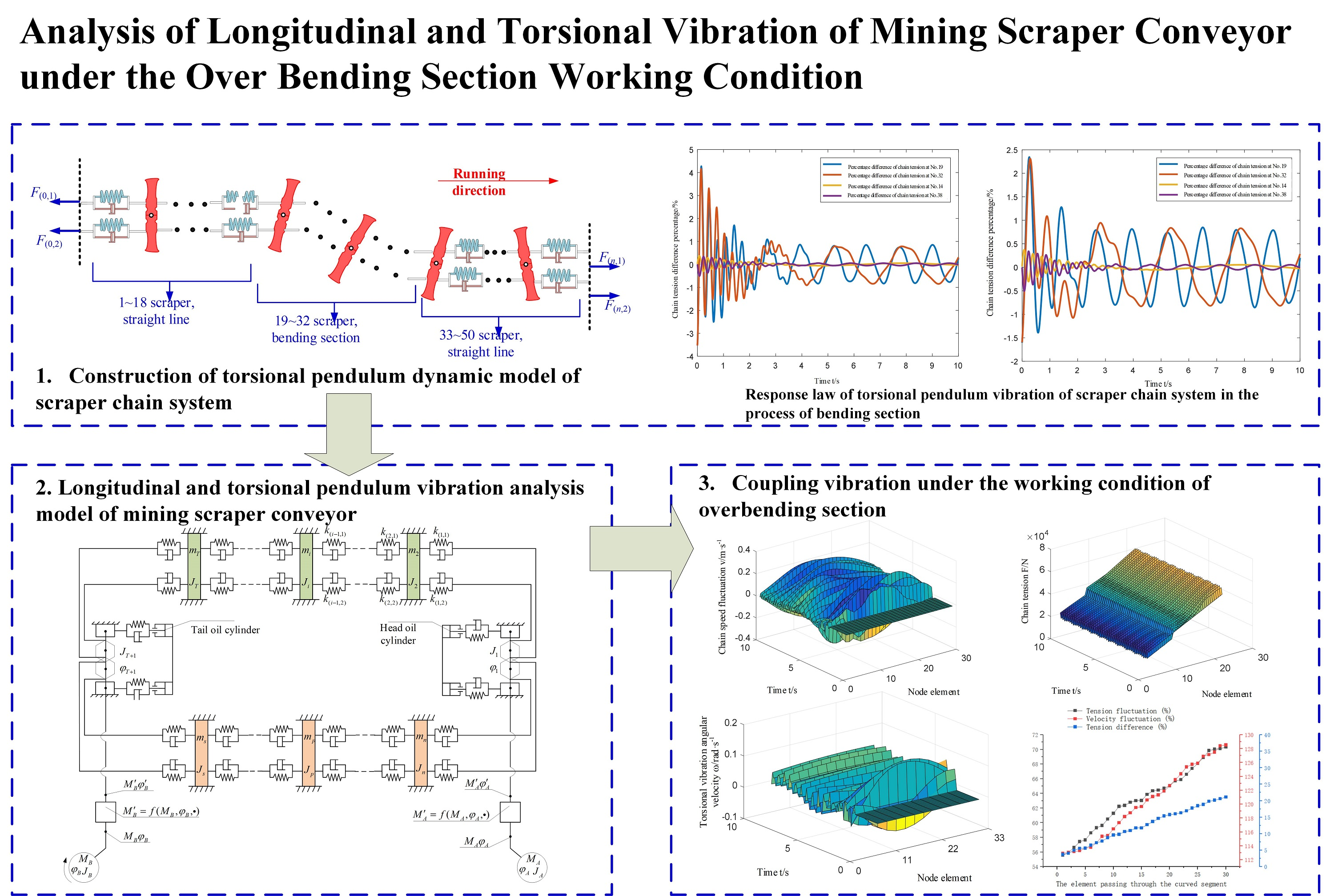#### Highlights

• The mechanical properties of about 10 scrapers before and after the scraper conveyor will be affected by the bending section
• When the scraper passes through the bending section, the speed will fluctuate by more than 10%
• The chain tension will fluctuate by more than 50%, and the tension difference between the two chains will exceed 5%

## 1. Introduction

The working process of mining scraper conveyor is affected by many factors, such as environment, working condition, fault and so on.  The external excitation of mining scraper conveyor mainly includes three aspects: The moving resistance between scraper and material is the load excitation, the friction and collision force between scraper and the middle slot along the conveyor line is the line shape excitation, and the impact force caused by chain clamping accident is called chain fault excitation [2-3].

Dolipsk M.  established the dynamic model of scraper conveyor under non-uniform load and obtained the slip rate of motor in this state. Wang  designed the dynamic tension test and intelligent coordinated control system of scraper conveyor, which realized the coordinated control of driving sprocket of scraper conveyor under heavy load. Xia  established the interaction analysis model of bulk coal scraper conveyor by discrete element method to predict the wear of scraper conveyor chute in the process of transportation. Zhang  established the virtual prototype model of the double-chain traction chain drive system, and analyzed the meshing characteristics of the drive sprocket and the tension change of the scraper chain. Jiang [8-9] proposes a monitoring method for the dynamic characteristics of the scraper conveyor on the basis of the speed difference between the head and the tail sprockets. Zhang  introduced a method to estimate the tension distribution of chain drive system of scraper conveyor based on multi-body dynamics theory and linear state observer. The multi-body dynamics model of chain drive system was constructed by ADAMS dynamic design software, and the mathematical model of chain drive system was established and solved by MATLAB function. By comparing the simulation results, the system performance of the dynamic model was verified.

Some experts and scholars have done a lot of work on flexible body dynamics, bending, finite element simulation and so on, which provide a theoretical basis for this study, such as references [11-16].

However, at present, no one has studied the torsional pendulum vibration characteristics of the scraper conveyor, especially the torsional pendulum vibration of the scraper when it passes through the bending section.

So, in this paper, the influence area and attenuation characteristics of torsional pendulum vibration under overbending conditions are studied. According to the influence area and attenuation characteristic of torsion pendulum vibration under different working conditions, the maximum number of scraper plates of torsion pendulum vibration units in different sections along the route is determined on the premise of solving reliability and precision, and a torsion pendulum vibration abbreviated model is constructed. It provides the foundation for the solvability of the vibration characteristic model of torsion pendulum of mining scraper conveyor.

## 2. Construction of torsional pendulum dynamic model of scraper chain system

Firstly, the Voigt model and the point-to-point tension method are used to establish the torsional pendulum vibration analysis model of the scraper chain system. Due to the special structure of the chain, the chain link cannot bear the pressure, but can only bear the tension. Therefore, the stiffness coefficient $k$ of each element can be expressed as Eq. (1):

1
${k}_{i-1}=\left\{\begin{array}{cc}k,& {x}_{i-1}\ge {x}_{i},\\ 0,& \mathrm{o}\mathrm{t}\mathrm{h}\mathrm{e}\mathrm{r}\mathrm{s}.\end{array}\right\$

In order to establish the dynamic model of torsional vibration of scraper chain system, the following assumptions need to be made. At the beginning of the study, the scraper chain has a certain initial tension. The mass of the chain is distributed to the scraper, but the moment of inertia of the scraper is not affected. The stiffness of the middle slot is very large, so it will not vibrated. The influence of the stiffness difference of the chain caused by structural factors on the torsional vibration of the scraper chain system is ignored.

Taking the scraper as the division node, the quality of the chain is allocated to the scraper, and the n scraper in the research area is divided into n nodes. The Voigt model is used to connect the nodes. At the same time, considering the friction between the chain scraper and coal, the friction between the chain scraper and the middle slot bottom, and the friction between coal and coal and the middle groove bottom, then the dynamic model of the scraper and chain system is shown in Fig. 1.

Fig. 1Analysis model of torsional vibration system of scraper chain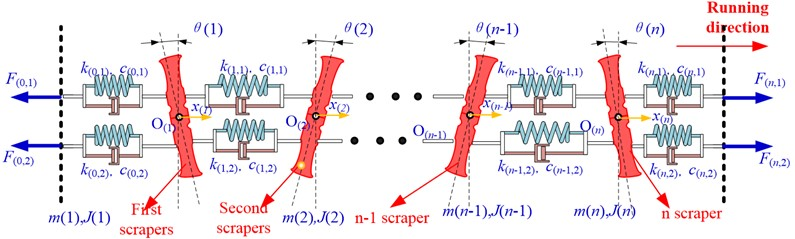In this case, translational $x\left(i\right)$ and rotational coordinates $\theta \left(i\right)$ are expressed by general $x$, that is, the generalized coordinate $x\left(i\right)$. In this way, it is convenient to solve the equation later, and the hand analysis of the $i$th scraper is carried out as shown in Fig. 2.

In the picture, $i$ denotes the $i$th scraper; $x\left(i\right)$ is the translation displacement of the $i$th scraper, the direction is positive to the right, that is, the coordinate system of the moving direction of the scraper is positive; $x\left(i+1\right)$ is the translation speed of the $i$th scraper m/s, set the direction to the right as positive; $x\left(i+2\right)$ is the rotation angle theta for the $i$th scraper $\theta \left(i\right)$, rad. set clockwise to positive; Set the clockwise positive for the angular speed of rotation of the $i$th scraper, that is $x\left(i+3\right)$, rad/s. ${F}_{j}\left(i,1\right)$ represents the static tension of the first branch of a ring chain that behind the $i$th scraper (a chain near the side of the coal wall) ${F}_{j}\left(i,2\right)$ represents the static tension of the second branch of a ring chain that behind the $i$th scraper (a chain near the side of the coal wall). The initial time of the scraper chain system is a stable state, the state of each scraper is considered as the coordinate origin of each scraper motion. At the beginning of the scraper chain system, there is a little pretension, also known as static tension, inside each circular chain strip. Static tension overcomes the friction resistance of material and scraper, and makes each scraper of the scraper chain system move steadily and uniformly. $W\left(i\right)$ is the $i$th scraper’s resultant external force arose by the chain, broken chain, over-bending section, loading materials and so on, which is named, $N$. Set The positive direction of external force $W\left(i\right)$ is the same as the running direction of the scraper. Let the external force distance acting on the $i$th scraper be $FM\left(i\right)$. The torque is positive if clockwise and negative if counterclockwise. ${F}_{d}\left(i,1\right)$ represents dynamic tension of the first branch of a ring that behind the $i$th scraper (a chain near the side of the coal wall) ${F}_{d}\left(i,2\right)$ represents dynamic tension of the second branch of a ring that behind the $i$ scraper (a chain near the side of the coal wall). Dynamic tension is the fluctuating value of tension when the scraper chain system is disturbed by the outside world and destroys the original equilibrium state.

Fig. 2Analysis model of torsional vibration system of scraper chain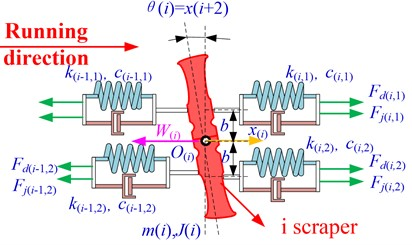## 3. Analysis of the influence area of torsional pendulum vibration under different working conditions

### 3.1. Definition of evaluation index for vibration stress wave attenuation of torsion pendulum

In the vibration process of scraper torsion pendulum, if each scraper and its connecting chain is regarded as a unit, it will make the model difficult to solve. The distribution, fault excitation state, parameters and chain dynamic tension along the freight load excitation line have the characteristics of time-varying and non-uniform along the line, resulting in the pace-time effect of conveyor torsional pendulum vibration transmission characteristics, The determination of the maximum number of scrapers in different sections along the conveyor is the key to the construction of the torsional vibration abbreviated model of the scraper chain system and the key to the numerical solution of the model.

In this paper, a self-defined evaluation index of vibration stress wave attenuation of torsion pendulum is defined. The index is used to evaluate the influence range of torsion plate around the action point of torsion pendulum excitation by torsional pendulum excitation, when the attenuation of stress wave of torsion vibration of a scraper is lower than the set evaluation index. It is considered that the scraper is not affected by torsional pendulum excitation, and can be divided into the outside of the torsional pendulum vibration influence area. The evaluation index of vibration stress wave attenuation of torsion pendulum is as Eq. (2):

2
$A{t}_{i}=\frac{\left|{F}_{z\left(i,1\right)}-{F}_{z\left(i,2\right)}\right|}{{F}_{z\left(i,1\right)}+{F}_{z\left(i,2\right)}}×100\mathrm{%}.$

The molecules represent the tension difference between the two chains at the left end of the $i$ scraper. The denominator represents the combined tension of the two chains at the left end of the $i$th scraper. Torsional vibration stress wave attenuation evaluation index is shown in Fig. 3.

The operating conditions of the scraper over bending section will cause the fluctuation of the running speed of the scraper chain system, the vibration of the scraper torsion pendulum and the fluctuation of the chain tension before and after the scraper. According to the custom index of torsional vibration stress wave attenuation, various working conditions of scraper chain system torsional vibration stress wave attenuation degree is evaluated, and the paper considers that the evaluation index is less than 0.5 % in the attenuation of torsional vibration of torsional vibration excitation points in front of or behind the $i$th scraper, and under this kind of condition, the scrapers after the $i$th scraper are not affected by torsional vibration excitation. In the subsequent study of the torsional vibration characteristics of the mining scraper conveyor, the scraper in the affected area can be regarded as a whole, and it is considered that the residual scraper is not affected by the torsion vibration, and can be divided into several units in average, and simplifies the dynamic model of the whole machine.

Fig. 3Torsional vibration stress wave attenuation evaluation index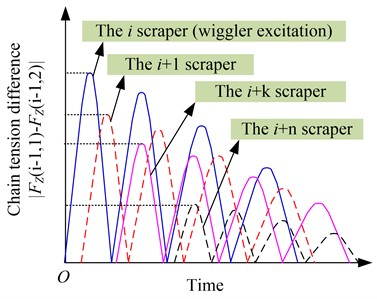a) Pendulum under the action of scraper chain tension difference diagram of decay process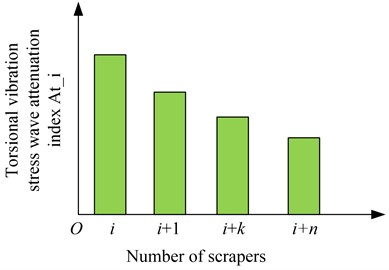b) Torsion vibration attenuation of stress wave diagram of evaluation index

### 3.2. The working condition of over bending section

When the mining scraper conveyor passes through the bending section, the scraper will collide with the middle slot, and the scraper will be subjected to extra bias load to make the scraper chain system torsional vibration. The torsional dynamic characteristics of scraper chain system under over bending condition are analyzed by using the torsional dynamic analysis model of scraper chain system established in this paper. This will be divided into two situations, the first is the case where the shearer goes up. In this case, the over-bending section of the scraper usually occurs behind the shearer running, because the speed of the scraper chain is higher than that of the shearer. In this case, it is equivalent to the over bending section of the no-load section, that is, the scraper chain of the over bending section is not subject to the material running resistance. The second is the downlink of the shearer, in which the over-bending section of the mining scraper conveyor occurs behind the shearer’s running rear, and in this case it is equivalent to the over bending section of the loaded section. That is, the scraper chain over the bending section will be subjected to material running resistance. The numerical calculation flow is shown in Fig. 4.

Fig. 4Numerical calculation flow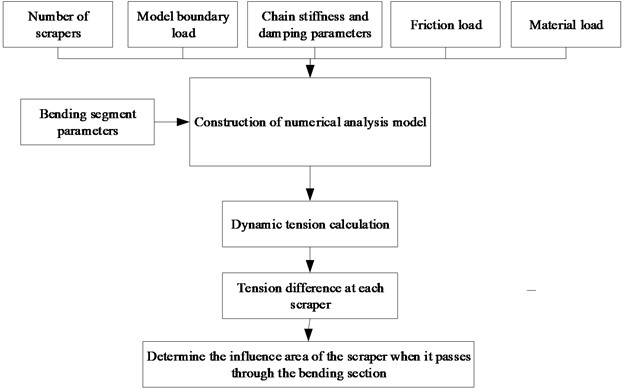There is no material at the front and rear of the bend section. Build the analysis model, as shown in Fig. 5. The number of scrapers in the scraper chain system is 50. It is set to simulate the over bending section of the chain at the 19-32 scraper, that is, 19, $j=$ 32.

Fig. 5Dynamic response analysis model of the scraper chain system in the process of bending section without material running ahead and the rear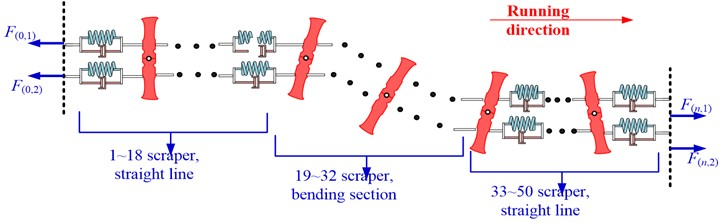Through numerically solving the dynamic response characteristics of the whole scraper chain system by MATLAB, the value of the additional load caused by the over bending section on the side of the scraper can be figured out. At the same time, in order to study the effect of the over-bending section of the scraper in different positions on the torsional vibration, the work conditions of the bending section occurred in the head, the middle of the fuselage and the tail are studied respectively, and the simulation results are shown in Fig. 6.

According to the custom index of torsional vibration stress wave attenuation, scraper chain system torsion vibration stress wave attenuation degree under the bending section is evaluated, when there is no material around during the operation.

If the torsional pendulum vibration of the 42nd scraper in front of the torsional pendulum excitation point and the 11th scraper in the rear attenuates to 20 % of the torsional pendulum vibration excitation point, then it is considered that under such working conditions, the influence area of torsional pendulum vibration is $\left[i-8,j+10\right]$. In the follow-up study of the torsional pendulum vibration characteristics of the scraper conveyor, the scraper whose influence area is $\left[i-8,j+10\right]$ can be studied as a whole. And it is considered that the residual scraper is not affected by torsion vibration. And it can be divided into several units in average, which simplifies the dynamic model of the whole machine.

Fig. 6Dynamic response of the scraper chain system in the process of bending section without material running ahead and the rear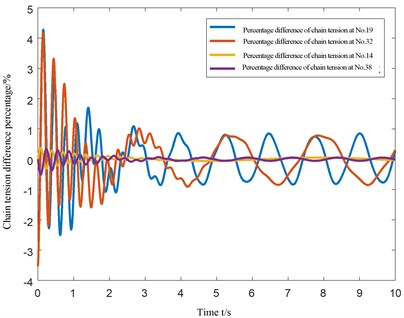Material working condition in front and rear of overbending section. Build the analysis model, as shown in Fig. 7. The number of scrapers in the scraper chain system is 50. Set at the 19th-32nd scraper to simulate the chain bending section.

Fig. 7Dynamic response analysis model of the scraper chain system in the process of bending section with material running ahead and the rear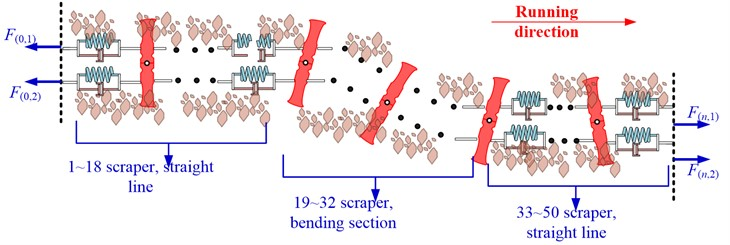The dynamic response characteristics of the whole scraper chain system are numerically solved by MATLAB, and the simulation results are shown in Fig. 8.

According to the custom index of torsional vibration stress wave attenuation, scraper chain system torsion vibration stress wave attenuation degree under the bending section is evaluated, when there is no material around during the operation.

If the torsional pendulum vibration of the 38th scraper in front of the torsional pendulum excitation point and the 14th scraper in the rear is attenuated to 0.5 % of the torsional pendulum vibration excitation point, then it is considered that under such working conditions, the influence area of torsional pendulum vibration is $\left[i-5,j+6\right]$. In the follow-up study of the torsional pendulum vibration characteristics of the whole scraper conveyor, the scraper whose influence area is $\left[i-5,j+6\right]$ can be studied as a whole. And it is considered that the residual scraper is not affected by torsion vibration, and can be divided into several units in average, which simplifies the dynamic model of the whole machine. The results show that the torsion vibration of the scraper chain system will be caused by the process of over-bending, and the torsion vibration is more obvious when there is no material at the beginning, that is, the influence area is larger, and the equivalent model contains more scrapers.

Fig. 8Dynamic response of the scraper chain system in the process of bending section with material running ahead and the rear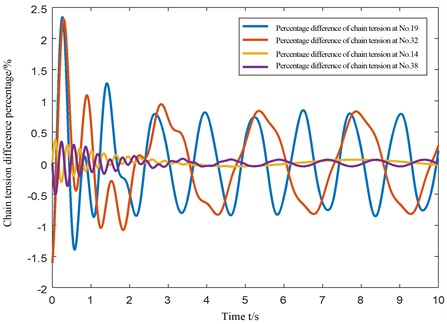## 4. Equivalent model of torsional pendulum vibration in over-bending section

The influence area of torsional pendulum vibration characteristics of material loading process is obtained in the previous paper to construct the equivalent model of torsion pendulum vibration, which is shown in Fig. 9.

The dynamic response analysis of scraper chain system under over-bending condition is carried out by applying the same load as the model without equivalent load, and the displacement and tension fluctuation of the two models relative to the scraper at the joint is analyzed and compared. Compared with the displacement and tension fluctuation trend of the scraper at the joint is basically the same. It can be considered that the analysis results of the equivalent model can replace the analysis model before the equivalent, which simplifies the degree of freedom of the model and reduces the amount of calculation.

Fig. 9Dynamic response analysis equivalent model of the scraper chain system in the process of the bending section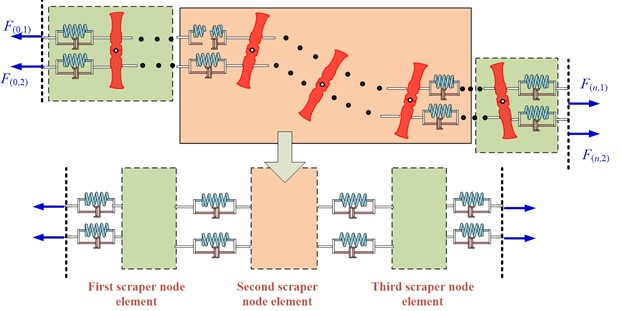## 5. Longitudinal and torsional pendulum vibration analysis model of mining scraper conveyor

By using finite element method, the double chain transmission system of mining scraper conveyor is divided into several units, each unit is connected by Kelvin-Voigt model, and the longitudinal discretization dynamic model of chain transmission system considering torsion pendulum excitation is established, as shown in Fig. 10.

Fig. 10Longitudinal dispersion dynamic model of the chain transmission system with considering the effect of incentive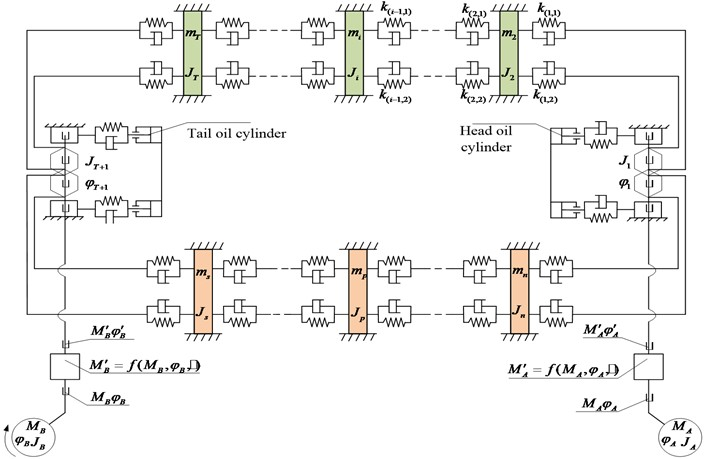Fig. 11Longitudinal discretization simplified dynamic model of chain transmission system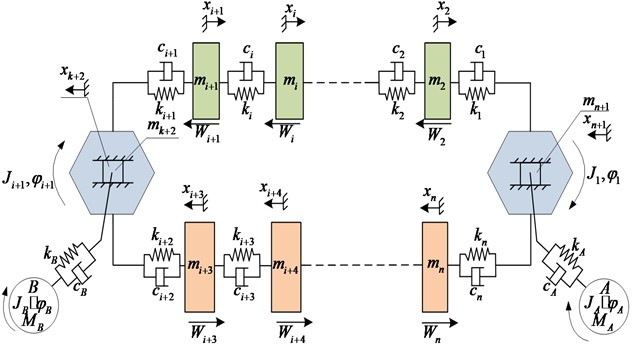This paper does not consider the torsion vibration of the scraper chain system caused by structural factors such as chain and sprocket, so the model of Fig. 10 can be further simplified, as shown in Fig. 11.

In order to simplify the calculation model, on the basis of the research results of Chapter 3, according to the different influence area and attenuation characteristic of torsion pendulum vibration excitation, the reliability and accuracy of the solution are the premise of establishing the torsional pendulum vibration analysis model. Determining the maximum number of scrapers in the unit section where the torsion pendulum vibration excitation action point is located, and considering that only the torsional pendulum vibration action point is located within the element section, and that the torsional pendulum vibration stress wave will not affect the scraper outside the element section, and make it produce torsional vibration, but the stress wave of the torsion pendulum vibration will make the scraper outside the element section produce longitudinal vibration along the line. Based on the longitudinal discretization dynamic model of chain drive system considering torsion pendulum excitation shown in Fig. 10, a dynamic differential equation is established as Eq. (3):

3
$\left\{\begin{array}{l}{J}_{A}{\stackrel{¨}{\phi }}_{A}+{k}_{A}\left({\phi }_{A}-{\phi }_{1}\right)+{c}_{A}\left({\stackrel{˙}{\phi }}_{A}-{\stackrel{˙}{\phi }}_{1}\right)={M}_{A},\\ {J}_{1}{\stackrel{¨}{\phi }}_{1}+{k}_{A}\left({\phi }_{1}-{\phi }_{A}\right)+{c}_{A}\left({\stackrel{˙}{\phi }}_{1}-{\stackrel{˙}{\phi }}_{A}\right)+\left({{F}_{1}}_{\left(t\right)}-{{F}_{n}}_{\left(t\right)}\right){R}_{A}=0,\\ \frac{d\left({m}_{2}{\stackrel{˙}{x}}_{2}\right)}{dt}-{F}_{1}\left(t\right)+{F}_{2}\left(t\right)+{W}_{2}=0,\\ ⋮\\ {J}_{B}{\stackrel{¨}{\phi }}_{B}+{k}_{B}\left({\phi }_{B}-{\phi }_{i+2}\right)+{c}_{B}\left({\stackrel{˙}{\phi }}_{B}-{\stackrel{˙}{\phi }}_{i+2}\right)={M}_{B},\\ {J}_{n}{\stackrel{¨}{\phi }}_{n}+{k}_{B}\left({\phi }_{i+2}-{\phi }_{B}\right)+{c}_{B}\left({\stackrel{˙}{\phi }}_{i+2}-{\stackrel{˙}{\phi }}_{B}\right)+\left({F}_{i+2}-{F}_{i+1}\right){R}_{n}=0,\\ \frac{d\left({m}_{i+3}{\stackrel{˙}{x}}_{i+3}\right)}{dt}+{{F}_{i+3}}_{\left(t\right)}-{{F}_{i+2}}_{\left(t\right)}-{W}_{i+3}=0,\\ ⋮\\ \frac{d\left({m}_{n}{\stackrel{˙}{x}}_{n}\right)}{dt}+{{F}_{n}}_{\left(t\right)}-{{F}_{n-1}}_{\left(t\right)}-{W}_{n}=0.\end{array}\right\$

Among them:

${{F}_{1}}_{\left(t\right)}={k}_{1}\left({\phi }_{1}{R}_{1}-{x}_{2}-{x}_{n+1}\right)+{c}_{1}\left({\stackrel{˙}{\phi }}_{1}{R}_{1}-{\stackrel{˙}{x}}_{2}-{\stackrel{˙}{x}}_{n+1}\right),$
${{F}_{j}}_{\left(t\right)}={k}_{j}\left({x}_{j}-{x}_{j+1}\right)+{c}_{1}\left({\stackrel{˙}{x}}_{j}-{\stackrel{˙}{x}}_{j+1}\right),$
${{F}_{i+1}}_{\left(t\right)}={k}_{i+1}\left({x}_{i+1}-{\phi }_{i+2}{R}_{i+2}+{x}_{k+2}\right)+{c}_{i+1}\left({\stackrel{˙}{x}}_{i+1}-{\stackrel{˙}{\phi }}_{i+2}{R}_{i+2}+{\stackrel{˙}{x}}_{k+2}\right),$
${{F}_{i+2}}_{\left(t\right)}={k}_{i+2}\left({\phi }_{i+2}{R}_{i+2}+{x}_{k+2}-{x}_{i+3}\right)+{c}_{i+2}\left({\stackrel{˙}{\phi }}_{i+2}{R}_{i+2}+{\stackrel{˙}{x}}_{k+2}-{\stackrel{˙}{x}}_{i+3}\right),$
${{F}_{n}}_{\left(t\right)}={k}_{n}\left({x}_{n}-{\phi }_{1}{R}_{1}+{x}_{n+1}\right)+{c}_{n}\left({\stackrel{˙}{x}}_{n}-{\stackrel{˙}{\phi }}_{1}{R}_{1}+{\stackrel{˙}{x}}_{n+1}\right).$

The dynamic tension of the connection point chain in each unit section is the associated variable, to figure out the transient dynamic tension by solving the longitudinal expansion dynamic model, and take transient dynamic tension as the total tension of the two chains The twist vibration applied in the unit section where the torsional pendulum vibration is applied, to figure out the torsion vibration characteristics of the scraper chain system of the internal unit section. It is set that the torsional vibration is applied in the section of the $i$th scraper unit, then the dynamic model of the scraper chain system in the section of the $i$th unit is established as the dynamic model of the torsional swinging vibration established in the previous paper. The motion differential equation is established for the $i$th scraper in the vibration analysis model of the torsion pendulum of the scraper chain system as Eq. (4):

4

## 6. Coupling vibration under the working condition of overbending section

By using MATLAB to simulate the vibration velocity and tension fluctuation of each unit section of the mining scraper conveyor under the working condition of the over bending section and the torsional vibration excitation action of the torsional pendulum vibration, the torsional vibration of each scraper in the unit section is obtained. In the middle of the mining scraper conveyor line shape incentive, assuming that there is no material on the mining scraper conveyor. The numerical calculation flow is shown in Fig. 12.

Velocity fluctuations and tension fluctuations for each cell segment are shown in Fig. 13.

Fig. 12Numerical calculation flow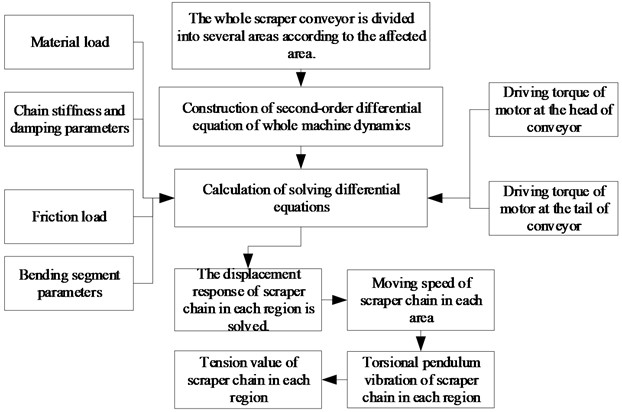Fig. 13The fluctuation of speed and tension under the excitation of the cargo unit section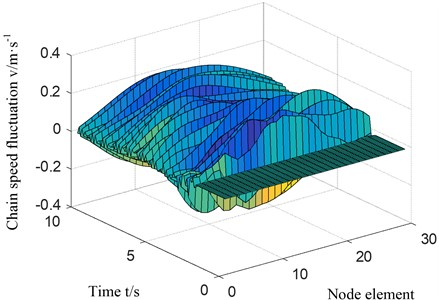a) Speed fluctuation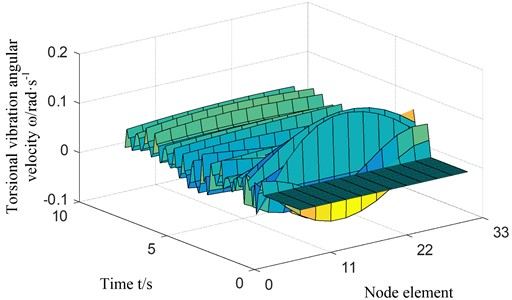b) Chain tension fluctuation

The angular velocity of the torsion pendulum vibration of each chain is shown in Fig. 14 within the section of the line form excitation unit.

Fig. 14Applying the cargo section incentive units, each chain of torsional vibration angular velocity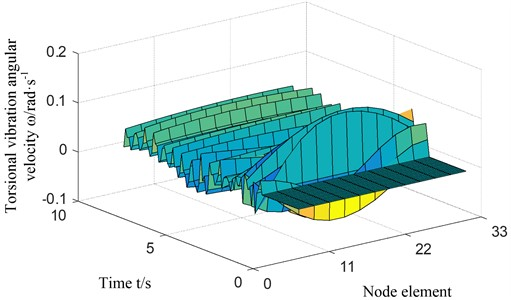According to the previous study, the first 10 and the last 8 scrapers are the torsional vibration affected areas in the applied line shape excitation, so there are 32 scrapers in the excitation unit section. Simulation result shows that the line form incentive will cause the longitudinal vibration of mining scraper conveyor, generating speed and the fluctuation of the chain tension, scraper speed and chain tension fluctuation is the most severe form, that is imposed at the line excitation, the maximum velocity fluctuations are caused by 113.8 % and 58.6 % of the tension fluctuation. On line configuration unit section line in the form of incentive system will cause the scraper chain scraper torsion vibration, resulting in internal scraper chain system of two chain tension fluctuations, The maximum difference in tension between chain 1 and chain 2 is 6.5 %.

In order to better grasp the tension and torsional pendulum vibration of the scraper chain when the scraper in different positions passes through the bending section, the response of the scraper passing through the bending section in the first to the 30th area is studied according to the above numerical simulation method, as shown in Fig. 15.

Fig. 15Dynamic response of scrapers at different positions when passing through the bending section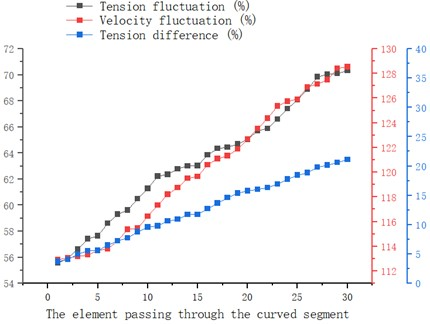The Abscissa in the picture represents different scraper areas, and the higher the value, the closer to the tail of the scraper conveyor. The results show that the more the scraper enters the bending section near the tail, the more obvious is the torsional vibration and the fluctuation of velocity and tension produced by the scraper chain system. The process of over-bending will cause slight vibration and torsion of the scraper chain system, and the torsion vibration is more obvious when there is no material at the beginning. The vibration is the weakest in the case of front and rear materials.

## 7. Conclusions

1) Based on Voigt model and point-by-point tension method, the simplified model of torsional pendulum vibration of scraper chain system is established, the law of scraper group vibration and chain tension change under the condition of scraper bending is studied, the influence area and attenuation characteristics of torsional pendulum vibration are determined, and the longitudinal and torsional pendulum vibration characteristics of scraper conveyor are obtained.

2) Under the working condition of the over-bending section of the scraper, when there is no material at the front and rear of the excitation, the range of influence is from the first 8 scrapers to the last 10 scrapers at the excitation. When there is no material at the front and rear of the excitation, the range of influence is from the first five scrapers to the last six scrapers at the excitation.

3) The research results of the overall dynamic characteristics of the scraper conveyor show that when the scraper passes through the bending section, it will produce 113.8 % speed fluctuation and 58.6 % tension fluctuation, and the tension difference between the two chains reaches 6.5 %.

The conclusion of this paper provides a theoretical basis for better understanding the operation characteristics of scraper conveyor in coal mine.

#### Acknowledgements

The authors are grateful to the support from the Liaoning Provincial Department of Education Project (LJ2020QNL012) and the National Natural Science Fund in China (51904142).